# Noise Suppression Products/EMI Suppression Filters

Noise Suppression Basic Course Section 1
Chapter 1
Chapter 2
Chapter 3
Chapter 4
Chapter 5
Chapter 6
Chapter 6

## EMI suppression filters

### 6-4. How basic and actual characteristics differ for filters

The frequency characteristics of LC filters discussed earlier were theoretical basic characteristics, so there may be cases where actual characteristics differ. Knowing what factors cause actual and basic characteristics to differ can be useful in selecting parts and making skillful use of them. This section describes these factors.

#### 6-4-1. The way the filter actually works can differ substantially

##### (1) Actual characteristics of filters

As an example where a filter's actual ability to eliminate noise differs from the basic characteristics described earlier, Fig. 1 and Fig. 2 show the results of measuring power supply noise when a bypass capacitor is being used.

##### (2) Test circuit

Fig. 1 shows a test circuit. A clock oscillator circuit operating at 20MHz is used as the noise source. The noise is drawn out along the power line, and then emitted from some 12cm diameter wiring formed into a loop. After installing a 1μF bypass capacitor on the power line to act as a filter, the change in noise was observed.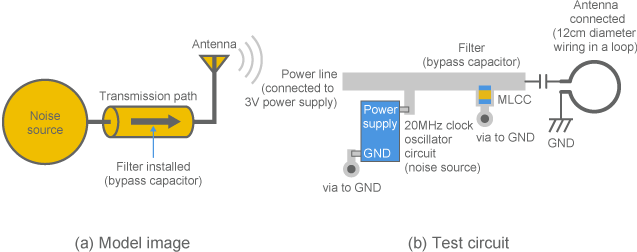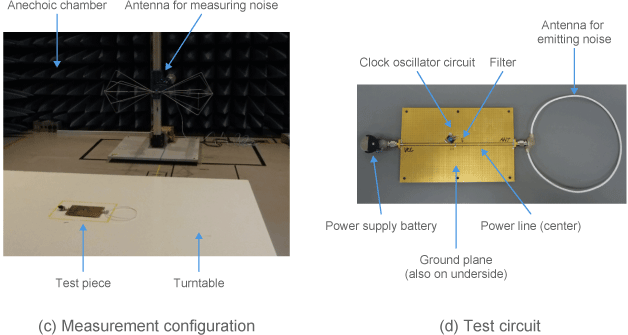Fig. 1 Test circuit in which a filter is used to eliminate noise

##### (3) Prior to using filter

Fig. 2 shows the measurement results. (a) shows the noise emitted when no filter is used. Strong noise was observed on nearly all frequencies in the 30 to 1,000MHz range that was measured.

##### (4) Not all capacitors have the same effect

(b) shows a three-terminal capacitor, as an example of how an ideal filter would function. The graph shows that noise emission is thoroughly suppressed at all frequency ranges, and that the amount of noise is so low that it can barely be observed.
(c) shows a case using an MLCC (a widely used bypass capacitor) , as an example of an implementation that is comparatively close to the ideal. The graph shows that its ability to eliminate noise suffers significantly compared to a three-terminal capacitor. However, noise is suppressed at all frequencies.
(d) shows a case where the same MLCC is used, but where a problem was intentionally introduced into the installation: an extremely long lead was attached. The graph shows a much weaker noise suppression effect.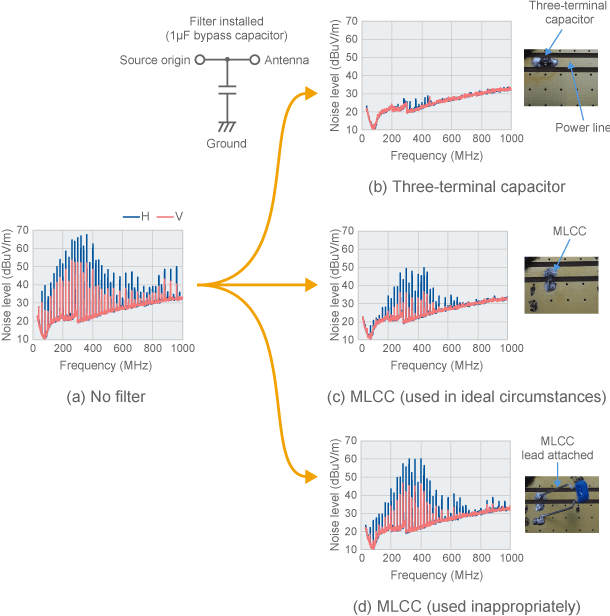Fig. 2 Results of measuring emitted noise

##### (5) Noise should not be visible, but...

The filter circuits used in examples (b) through (d) are the same as far as the circuit diagram is concerned: a 1μF bypass capacitor. They should therefore all function as low-pass filters with 20dB/dec. slopes, and their basic characteristics should be exactly the same as in Section 6-3-2.
Fig. 3 shows the basic characteristics of a 1μF capacitor. There is an insertion loss of at least 70dB in the frequencies above 30MHz measured in Fig. 2. In other words, the noise observed in Fig. 2 (a) should not be visible on the graph, assuming the capacitor is functioning properly.
However, Fig. 2 shows that, when actually measured, there are cases where noise remains—and that the level varies significantly depending on what parts are used. It is therefore important to confirm the actual characteristics when suppressing noise.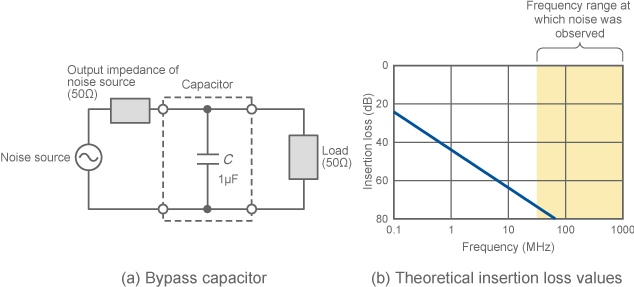Fig. 3 Insertion loss with a 1uF capacitor (theoretical values)

##### (6) Factors causing actual characteristics to differ from basic characteristics

In this way, the actual characteristics of filters differ from basic characteristics. The various factors involved can be split into two categories: factors caused by the good or poor performance of the filter itself, and factors caused by interaction between the filter and something external to it.
First, the noise reduction effect of the filter itself differs from the basic characteristics. This will be covered in the next section. Second, the interaction between the filter and something external to it can have an effect on the filter's actual characteristics. The latter can change in a variety of ways depending on the conditions in which the filter is being used. It is therefore extremely difficult to accurately estimate a filter's actual characteristics from a theoretical standpoint.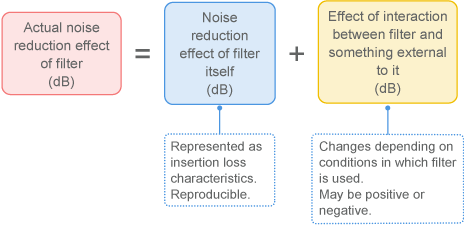Fig. 4 Analysis of actual characteristics of filters

#### 6-4-2. A filter's effectiveness differs from its basic characteristics

Fig. 5 shows comparisons between the frequency characteristics and the theoretical values of a simple capacitor and inductor, as an example of where a filter's actual characteristics differ from its basic characteristics.
As mentioned in Section 6-3, capacitors and inductors are used as elements for LC filters. Fig. 5 shows the results of re-editing the data introduced in 6-3-7, and then comparing the measured insertion loss against the theoretical value. Actual characteristics clearly diverge significantly from theoretical values at frequencies at and above 100MHz.
This will not be apparent when simply looking at the electrostatic capacitance or inductance of these parts, in regions showing frequency characteristics that differ from theoretical values. The actual characteristics of capacitors and inductors will be introduced in the next sections. The explanation will begin with a slightly detailed discussion of capacitors, and will then use this information as a foundation to cover inductors and LC filters.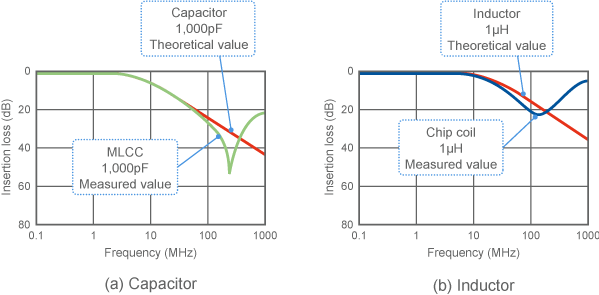Fig. 5 Actual insertion loss characteristics of capacitors and inductors

Top of page

### How basic and actual characteristics differ for filters" - Key points

• The actual noise reduction effect of an LC filter differs from its basic characteristics.
• The characteristics of capacitors and inductors used to create LC filters ultimately differ from the theoretical values.